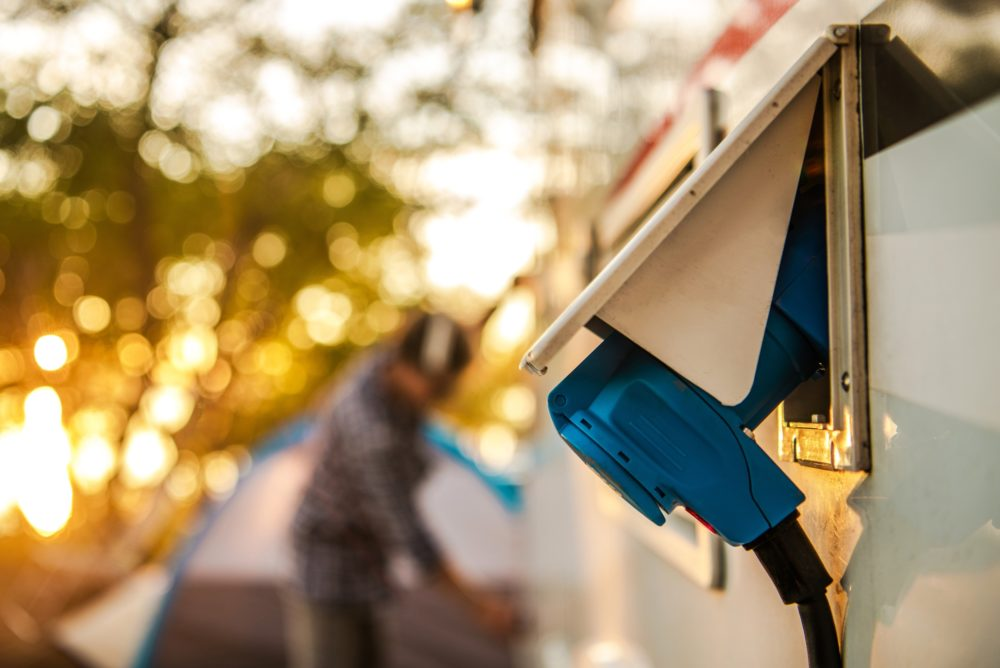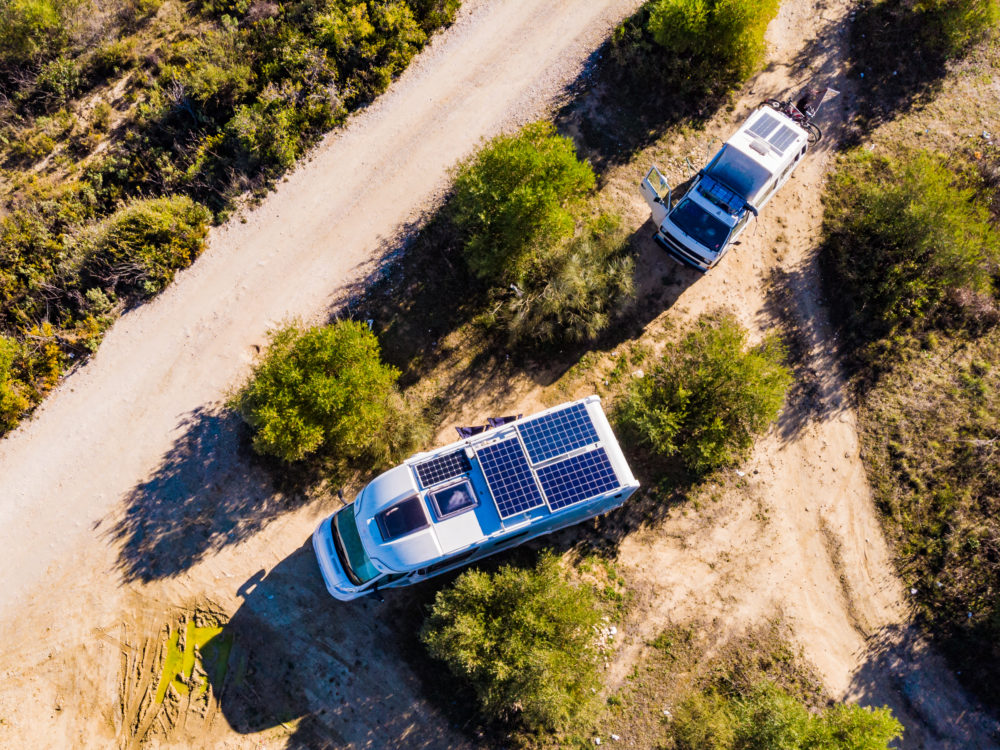# How Many Watts Does An RV AC Use?

## Understanding Power Vs. Energy

There is a difference between power and energy, although many often use these terms synonymously.Power is measured in Watts.  Watts = volts X amps which can also be expressed as watts/volts = amps. Therefore, if you have determined your RV typically doesn’t need more than 2,100 peak watts of shore power, you can plug into a 20-amp electrical outlet without worry (2,100 watts / 120 volts = 17.5 amps)

The RV has two or three different electrical systems: a 12V DC system, a 110V AC system, and for driveables (Class A-C), a 120V AC coach system.

Energy is the amount of power used over a specific period of time, measured in kWh.

Ideally, most RVs use up to 150 watts of energy per hour, which is equivalent to around 20 kWh per day. When it comes to other appliances, an AC unit will use up to 1000 KWh per year or 120 kWh each day. An electric space heater uses about 1500 – 2000 KWh annually or 250 kWh every 24 hours.

### Relationship Between Energy And Power

Energy is measured at the electric meter or can be calculated depending on per-appliance draw/usage, while power is the amount of Watts required to start up and run each specific appliance.

Below is an example that shows how one can calculate the conversion from power to energy:

“Much like one kilowatt is equal to 1,000-watts of power, one kilowatt-hour is equivalent to 1,000-watts, or joules, of energy use over one hour. If you wanted to convert watts into kilowatt-hours to find out how much energy your coffee maker consumes each day, you would multiply the power consumption in watts by the number of hours used. Then, divide that number by 1,000.

Here’s the Formula for Calculating Watts Into Kilowatt-Hours:

• kWh = (watts × hrs) ÷ 1,000

For example, to find the kWh of 1,200 watts for 3 hours:

• kWh = (1,200 × 3) ÷ 1,000
• kWh = 3,600 ÷ 1,000
• kWh = 3.6

Suppose you were interested in doing the reverse to determine how many watts your coffee maker is, based on its kilowatt-hours. In that case, you could easily do so by making a few simple adjustments to the formula.

For this conversion, multiply the energy used in kWh by 1,000 to find the energy consumption in watt-hours. Then, you must divide that number by the number of hours you used it.

Here’s the Formula for Calculating Kilowatt-Hours Into Watts:

• watts = (kWh × 1,000) ÷ hrs

For example: let’s find the watts of power for 3.6 kWh of energy used in 3 hours.

• watts = (3.6 kWh × 1,000) ÷ 3 hrs
• watts = 3,600 ÷ 3 hrs
• watts = 1200 W

### What’s the Difference Between Watts and Amps?

Watts and amperes, also known as amps, are both units used to measure electrical power consumption or production. All electronic devices have their energy consumption listed on their label in either watts or amps. If the label on your device lists amps, you can calculate the wattage by using a simple formula.

Here’s the Formula for Calculating Amps to Watts:

For example, if you have a 120V device with a 20 amp label, that’s equivalent to a wattage of 2400. In equation form, that is: 20A x 120V = 2400W”

(Source: justenergy.com)

## How Many Watts Does An RV AC Use?

For any appliance in the RV, the steps below show how to figure out the battery needs. When it comes to specific appliances, each appliance has a label that shows the amount of Watts it will draw.

1. List all your electrical devices/appliances
2. Figure out the daily draw of each of these
1. List each device’s power consumption (in Watts)
2. List the average use duration per device per day (in hrs)
3. Calculate the daily Watt-hours (W*hrs)
4. Divide that number by 12. This is your Amp-hour.
1. Calculate your battery size with lithium

Step 1: Take ‘daily energy usage’

Step 2: Double it

Step 3: Round up to nearest 100Ah

1.  Calculate your battery size with AGM

Step 1: Take ‘daily energy usage’

Step 2: Double it

Step 3: Double it, again

Step 4: Round up to nearest 100Ah

(Source: www.asobolife.com)

After you check the AC label, do some math:

For every 1,000 BTU cooling output, the RV AC will require about 100 watts of electric power input.

“All RV AC units run on a 115V circuit. This is the key information you will need to figure out how many watts does a 50 amp RV AC use, for example. To get the wattage, you need to multiply amps with the voltage. Example: How many watts does a 50 amp generator put out? Simple calculation: 50A×115V = 5,750 watts. Basically, a 50 amp generator is the same as a 5,750-watt generator.”

For those who don’t like to do this manually, there is a handy calculator online: learnmetrics.com

### Look Through A Specification Label

To locate the model number for your inside ceiling assembly, remove the plastic grille covering the assembly. The model number will be somewhere on the metal frame above the grille. This label is also the specification label and should hold the power needs specifications on it.

There are Websites like the one listed below that have useful Amps and Watt usage calculators: learnmetrics.com

### Using Energy Efficiency Ratio (EER)

EER stands for Energy Efficiency Ratio. The EER rating for an air conditioner is calculated by dividing the BTU (British Thermal Units) rating by the wattage. For example, a 12,000-BTU air conditioner that uses 1,200 watts has an EER rating of 10 (12,000/1,200 = 10).

(Source: westernhillsheating.com). )

A good EER rating is anything over 12.

### Using Electricity Usage Monitor

You want to track your electricity usage, especially when you are staying at an RV park that charges you for metered electric usage (in kWh).

Below is a video that shows you how to do this:

You can calculate your per-appliance usage, using a tool like Efergy Elite (costs anywhere between \$70-300). For total usage, you modify your “dog bone” plug to the pedestal, using the Efergy monitors to interrupt the circuit, so to speak.

The system has sensors that communicate with the interface unit every 10 seconds on real-time usage per appliance. You can see drains, surges and how much total you have left to use, if you budget.

### Using RV AC Wattage Chart

The chart below shows you the Wattage required for startup and then the average Wattage to keep the particular appliance running. A complete list can be found on the Website listed below.  If you look up your btu value on the AC label and it states it’s a 10,000 btu unit, this means that you need a 2,000 Watt initial draw and then, on average, 700 Watt available to keep the unit running.

Keep in mind that often, you have several appliances running simultaneously and they have a compound Wattage draw.

(Source: www.ramsond.com)## What Is The Difference Between Starting And Running Watts?

The starting Wattage is the amount needed to get the unit started, while the running Wattage is the average amount to keep the unit going. One thing that can help reduce the high startup Wattage draw is a “Soft Start” install, which reduces the high draw significantly.

### RV AC Amps Chart

Below is a partial example of average Amperage draws of an AC start-up and AC running, compared to other appliances:

Source: www.lilacresort.com

Below is a combined partial chart of Wattage and Amperage of different appliances:

Source: ilovervlife.com

## How Much Does It Cost To Run An RV AC Unit?

Of course, this varies greatly on the economy/cost of electricity, the state you are located in, the age of the RV and its condition, as well as the use. However, here is an example:

“The average draw for a Class A RV is around 430 watts. That means that an hour of AC usage will cost you about \$2.07 per hour in electricity costs, or \$25 per day if you’re running it 12 hours each day. Of course electric rates vary in all RV Parks. […]

Typically to run 15,000 BTU RV AC unit for an hour it will cost about \$0.07 on a thermally efficient AC system and about \$0.26 for an inefficient one – this difference in power usage can be quite significant considering that central air only runs to cool the entire RV while window units may need to be running all day long just cooling small areas of your home or RV at once.”

(Source: crowsurvival.com.)

## FAQs

### How many watts does a Coleman RV AC use?

This depends on the actual unit, but it is anywhere from 1075-1350 Watt.

### Will a 2000-Watt generator be good enough to run an RV AC generator?

Yes, it should be possible; however, one needs to consider any additional appliance draws on the generator.

### Can you use solar panels to run an RV AC?

“Yes, it’s technically possible to power an RV air conditioner with solar panel. But to generate enough power, a large amount of solar panels and upgrades to the electrical system are required. Or, another device called a “Soft Start.” The vast majority of RV owners will not go to these lengths.” I guess it’s a matter of upgrade budget. (Source: camperreport.com. )

### How long does an RV AC unit last?

“Even a new air conditioning unit will need to be replaced eventually, depending on factors like how often it is used and how well it’s maintained. So, how long do RV AC units last? An average RV AC unit lasts anywhere from 3 years to 5-10 years or longer.” (Source: www.jeffsetter.com.)

### Can you run your RV AC on battery power?

“The short answer is yes. With the proper equipment and enough batteries, you can run your RV AC unit on batteries. In addition to the lithium batteries, you’ll need an inverter to invert the DC battery power into AC power for most air conditioning units.”

(Source: battlebornbatteries.com.)

### Can you run your RV AC all night?

“Yes. You can run your RV air conditioner all night either from a generator or if you are connected to a 50 amp hookup where you are parked. And most generators can run your AC all night using very little gasoline.” (Source: rvparenting.com.)